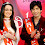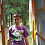# Class 10, CBSE (NCERT) Science | Chapter-1, Chemical Reactions and Equations | NCERT Solution - Science Textbook Exercise

## 10th NCERT (CBSE) - Science (Chemistry)

### Chapter 1, Chemical Reactions and Equations

#### NCERT Solutions of Science Textbook Chapter Exercise Questions

Question.1: Which of the statements about the reaction below are incorrect?
2PbO + C ---> 2Pb + CO
(b) Carbon dioxide is getting oxidized.
(c) Carbon is getting oxidized.
(d) Lead oxide is getting reduced.
(i) a and b (ii) a and c (iii) a, b and c (iv) all.

Question.2: Fe2O3 + 2Al ---> Al2O3 + 2Fe
The above reaction is an example of a
(a) combination reaction
(b) double displacement reaction
(c) decomposition reaction
(d) displacement reaction.

Question.3: What happens when dilute HCl is added to iron filings ? Tick the correct answer.
(a) Hydrogen gas and iron chloride are produced.
(b) Chlorine gas and iron hydroxide are produced.
(c) No reaction takes place.
(d) Iron salt and water are produced.

Question.4: What is a balanced chemical equation ? Why should chemical equation be balanced ?
Answer: An equation having an equal number of atoms of each element on both the sides is called a balanced chemical equation.
According to this law, mass can neither be created nor destroyed. In other words, the total mass of reactants should be equal to the total mass of the products in a chemical equation.  Therefore, the total number atoms of each element participating in the reaction must be equal on both sides of the chemical equation i.e. before and after reaction. Hence, it is for the above reason that a chemical equation should be balanced.

Question.5: Translate the following statements into balanced chemical equations.
(a) Hydrogen gas combines with nitrogen to form ammonia.
(b) Hydrogen sulphide burns in air to give water and sulphur dioxide.
(c) Barium chloride reacts with aluminium sulphate to give aluminium chloride and a precipitate of barium sulphate.
(d) Potassium metal reacts with water to give potassium hydroxide and hydrogen gas.
(a) N2 + 3H2 ---> 2NH3
(b) 2H2S + 3O2 ---> 2H2O + 2SO2
(c) 3BaCl2 + Al2(SO4)3 ---> 3BaSO4 + 2AlCl3
(d) 2K + 2H2O ---> 2KOH + H2

Question.6: Balance the following chemical equations.
(a) HNO3 + Ca(OH)2 ---> Ca(NO3)2  + H2O
(b) NaOH + H2SO4 ---> Na2SO4 + H2O
(c) NaCl + AgNO3 ---> AgCl + NaNO3
(d) BaCl2 + H2SO4 ---> BaSO4 + HCl
(a) 2HNO3 + Ca(OH)2 ---> Ca(NO3)2  + 2H2O
(b) 2NaOH + H2SO4 ---> Na2SO4 + 2H2O
(c) NaCl + AgNO3 ---> AgCl + NaNO3
(d) BaCl2 + H2SO4 ---> BaSO4 + 2HCl

Question.7: Write balanced chemical equations for the following reactions.
(a) Calcium hydroxide + Carbon dioxide ---> Calcium carbonate + Water
(b) Zinc + Silver Nitrate ---> Zinc nitrate + Silver
(c) Aluminium + Copper chloride ---> Aluminium chloride + Copper
(d) Barium chloride + Potassium sulphate --->. Barium sulphate + Potassium chloride
(a) Ca(OH)2 + CO2 ---> CaCO3 + H2O
(b) Zn + 2AgNO3 ---> Zn(NO3)2 + 2Ag
(c) 2Al + 3CuCl2 ---> 2AlCl3 + 3Cu
(d) BaCl2 + K2SO4 ---> BaSO4 + 2KCl

Question.8: Write the balanced chemical equations for the following and identify the type of reaction in each case.
(a) Potassium bromide + Barium iodide ---> Potassium iodide + Barium bromide
(b) Zinc carbonate ---> Zinc oxide + Carbon dioxide
(c) Hydrogen + Chlorine ---> Hydrogen chloride
(d) Magnesium + Hydrochloric acid ---> Magnesium chloride + Hydrogen
(a) 2KBr + BaI2 ---> 2KI + BaBr2
(b) ZnCO3 ---> ZnO + CO2
(c) H2 + Cl2 ---> 2HCl
(d) Mg + 2HCl ---> MgCl2 + H2

Question.9: What does one mean by exothermic and endothermic reactions? Give examples.
Exothermic reactions: Those reactions in which energy is released in the form of heat are called exothermic reactions. Examples -
(1) All combustion reactions e.g.
CH4 + 2O2 ---> CO2 + 2H2O + Heat
(2) Thermite reactions e.g.
2Al + Fe2O3 ---> 2Fe + Al2O3 + Heat
Combinations are generally exothermic in nature. The decomposition of organic matters into compost is an example of exothermic reaction.
Endothermic reactions: Those reactions in which energy is absorbed are called endothermic reactions. Examples -

,

also, the reaction of photosynthesis -

Question.10: Why is respiration considered an exothermic reaction? Explain.
Answer: For the sustenance of life we require energy. We obtain this  energy from our food. In the process of digestion, food molecules get converted into simpler substances such as, glucose. Then glucose combine with oxygen and provide energy to our body. The whole process is known as respiration. As because, energy is released during the process of respiration, so it is considered an exothermic reaction. For example,
C6H12O6 + 6O2 ---> 6CO2 + 6H2O + energy

Question.11: Why are decomposition reactions called the opposite of combination reactions? Write equations for these reactions.
Answer: Decomposition reactions are those reactions in which a compound is broken into two or more new compounds.

Combination reactions are those reactions in which two substances are combined to form a new substance.
CaO + CO2 ---> CaCO3 + energy
In above examples, both the reactions are same but show opposite directions, so decomposition reaction is called the opposite of combination reaction.

Question.12: Write one equation each for decomposition reactions where energy is supplied in the form of heat, light or electricity.
(a) Decomposition by heat or thermal energy

(b) Decomposition by light or photolysis
2AgCl ---> 2Ag + Cl2 (in presence of light)
(c) Decomposition by electricity or electrolysis

Question.13: What is the difference between the displacement and double displacement reactions? Write equations for these reactions.
Answer: In displacement reaction, one element from its salt is displaced by a more reactive element e.g. in following example, Cu is displaced by Zn from CuSO4 because Zn is more reactive.
CuSO4 + Zn ---> ZnSO4 + Cu
In double displacement reaction, exchange of ions takes place between two reactants to form new products.
Na2SO4 + BaCl2 ---> BaSO4 + 2NaCl

Question.14: In the refining of silver, the recovery of silver from silver nitrate solution involved displacement by copper metal. Write down the reaction involved.
Answer: When copper is mixed in silver nitrate solution, it displaces the silver because copper is more reactive than silver.
2AgNO3 + Cu ---> Cu(NO3)2 + 2Ag

Question.15: What do you mean by a precipitation reaction? Explain by giving examples.
Answer: The reactions in which a precipitate is formed are called precipitation reactions. Examples:
1. Na2SO4 + BaCl2 ---> BaSO4 + 2NaCl
In this reaction, BaSO4 is obtained as precipitate.
2. Na2CO3 + CaCl2 ---> CaCO3 + 2NaCl
Here calcium carbonate is obtained as precipitate.

Question.16: Explain the following in terms of gain or loss of oxygen with two examples each.
(a).Oxidation
(b).Reduction
(a) Oxidation: The reactions in which gain of oxygen takes place are called oxidation. Examples -
1. 2Cu + O2 ---> 2CuO
2. 2H2 + O2 ---> 2H2O
(b) Reduction: The reactions in which loss of oxygen takes place are called reduction. Examples -
1. ZnO + C ---> Zn + CO
2. CuO + H2 ---> Cu + H2O

Question.17: A shiny brown coloured element ‘X’ on heating in air becomes black in colour. Name the element ‘X’ and the black coloured compound formed.
Answer: The shinny brown colored element ‘X’ is copper. When it is heated in air, it becomes black due to the deposit of copper oxide.

Question.18: Why do we apply paint on iron articles ?
Answer: We apply paint on iron articles to prevent them from corrosion. Paint prevents iron from coming in contact with air and moisture. Thus, painting prevents rusting of iron articles.
Question.19: Oil and fat containing food items are flushed with nitrogen. Why ?
Answer: Nitrogen being an inert gas does not react easily with the oil and fat present in the food substances. But oxygen reacts easily with the food substances and makes them rancid.  It is because of this reason that food items containing oil and fat are flushed with nitrogen gas before their packing to remove oxygen present in the pack.

Question.20: Explain the following terms with an example each:
(a) Corrosion (b) Rancidity
(a) Corrosion: Corrosion is a process in which materials deteriorate as a result of a chemical reaction with moisture and other chemicals present in its surroundings.  Generally, metals get corroded more easily. For example, iron in the presence of moisture, reacts with oxygen  (air) to form hydrated iron oxide also called Rust.
4Fe + 3O2 +nH2O ---> 2Fe2O3.nH2O
(b) Rancidity: It is a process of oxidation in which fats and oils present in food items are oxidized that can be easily noticed by the change in their taste and smell.
Rancidity can be prevented by:
1. Storing food in refrigerators or in air-tight containers.
2. Flushing oil and fat containing food items with nitrogen before their packing.

#### Further Study on Chapter 1, Chemical Reactions and Equations

• Chemical Reactions and Equations | Class 10 NCERT (CBSE) Science (Chemistry) Textbook In-Text Questions - Answers
• Class 10 NCERT (CBSE) Science Chemistry - Multiple choice questions (MCQ) | Chapter 1,  Chemical Reactions and Equations
• Xth CBSE Chemistry | NCERT Science Textbook - Chapter 1, Chemical Reactions and Equations | Notes and Sample Questions-Answers

1.Very helpful....keep the good work going!!

2.oh problem solved...

3.thank ya!!! :)

4.thanks..............

5.thank uuuuuuuuuuuuu very much
keep it up

6.itz briliant ...........thnkzzzzzzzzz alot ....

7.THANX,MY PROBLEMS ARE SOLVED

8.9.my problem is solve for science exam

10.awesome my problem has solved

11.This comment has been removed by a blog administrator.

12.thanks it solved my problem!!! :)

13.thanks a lot !! really this site is helpful !! pls post answers of other chapter too as soon as possible :P :)

14.gud ..continue this service..

15.This comment has been removed by a blog administrator.